Next: Bibliography Up: Self Calibration Previous: Derivation of Kruppa's Equations

## Explicit Computation

To derive explicit expressions for the matrices D and D' in terms of the fundamental matrix F, let us reconsider the above argument. Let F=UWVt be the Singular Value Decomposition of F. Here, Uand V are orthogonal, and W is a diagonal matrix with diagonal values r, s, 0. We can write this as follows: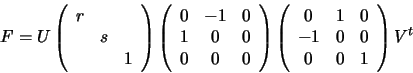Define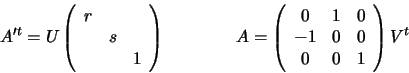Hence, F = A'tF'A with A,A' non-singular and F' having the desired canonical form. Applying the transformations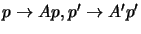and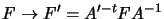, we see that p'Fp is unchanged and F becomes canonical, so A,A' are the required rectifying transformations. These transformations take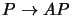,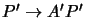and hence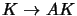,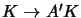, and hence the DIAC C=KKt becomes respectively D=ACAt and D'=A'CA't.

Now explicitly compute the dij in order to use equation (5.5). Decompose A and A' by rows: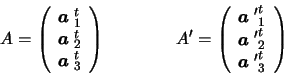Then, D= ACAt implies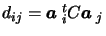, and we have the following explicit form for the Kruppa equations: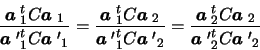(5.6)

We can write these equations directly in terms of the SVD of the fundamental matrix.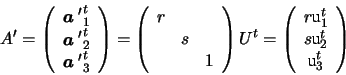where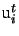is the i-th column of U. And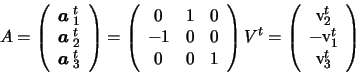where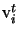is the i-th column of V. From (5.6) we obtain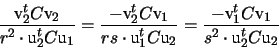Our problem has five degrees of freedom. Each pair of images provides two independent constraints. From three images we can form three pairs which provide three pairs of constraints. This is enough to solve for all the variables in C. However, note that all of the equations are multivariable quadratics in the coefficients of C. This makes the problem quite painful to solve in practise.

The difficulty of such purely algebraic approaches explains why alternative approaches have been explored for self calibration.  provides one such alternative. In any case, an algebraic solution can only ever provide the essential first step for a more refined bundle adjustment (error minimization) process.Next: Bibliography Up: Self Calibration Previous: Derivation of Kruppa's Equations
Bill Triggs
1998-11-13